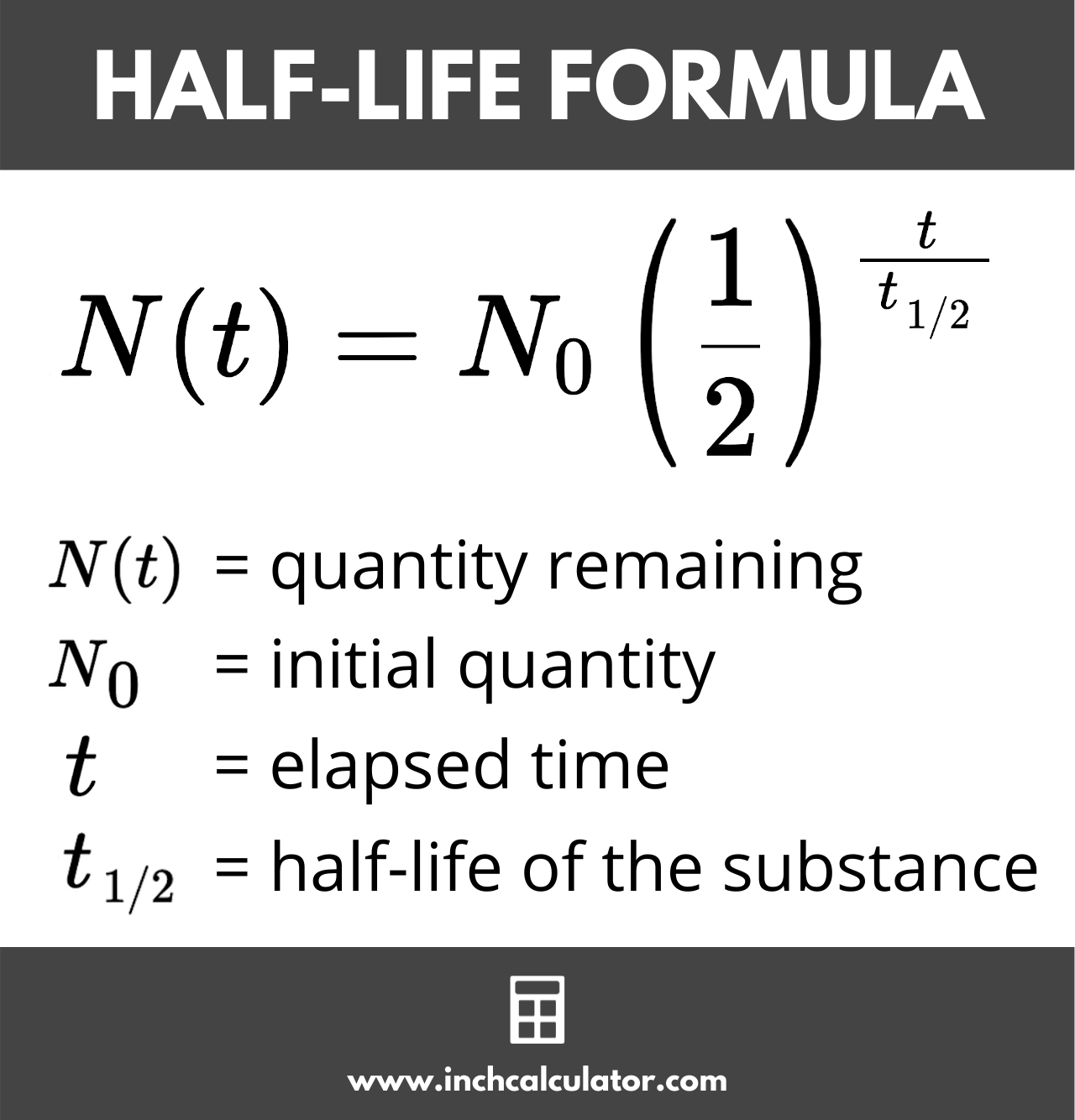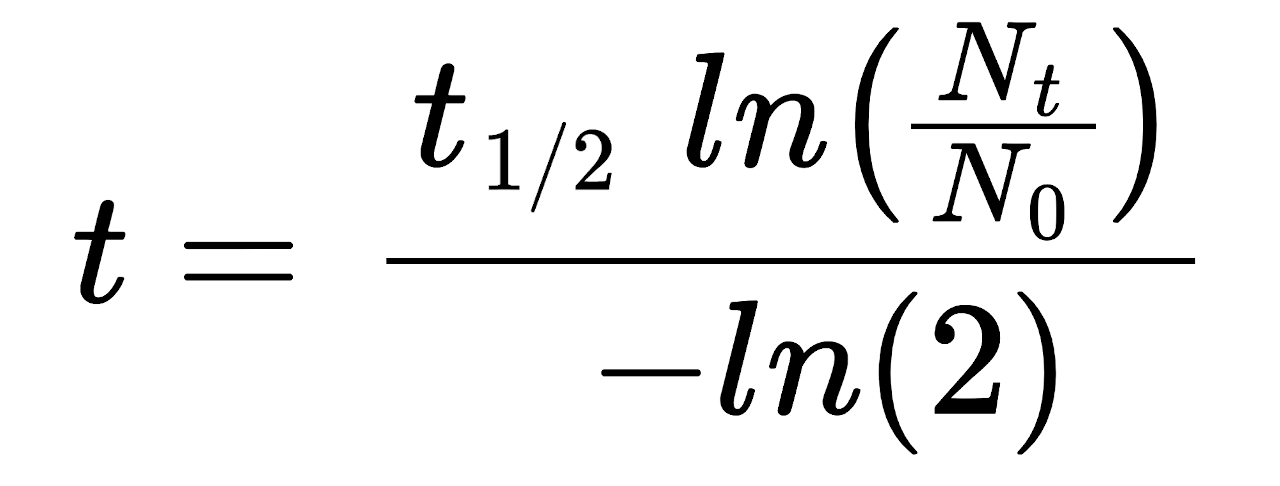# Half-Life Calculator

Calculate half-life, elapsed time, initial, or remaining quantity using this easy calculator. Read below to learn the half-life formula and how to solve it.

Enter one of the following:
Enter one of the following:
Enter one of the following:

## Half-Life:

seconds

### Decay Constant

seconds

seconds
Learn how we calculated this below

## What is Half-Life?

Half-life is the time needed to reduce the quantity of a substance to half its initial amount. It is commonly used to define the decay of radioactive material, where half-life is the amount of time needed for half the atoms of a particular isotope to decay.

A radioactive isotope is an atom that has an unstable nucleus, giving off radiation and emitting various kinds of particles until it reaches a stable state. There are several kinds of particles that can be emitted, including:

• Alpha particles – a helium nucleus
• Beta particles – electrons or positrons
• Gamma particles (aka gamma rays) – photons
• Neutrons – high speed particles

Loss of alpha particles or beta particles changes the number of protons in the nucleus, which changes the atom to a different element. For example, uranium decays to thorium through loss of an alpha particle. The new atom may also be unstable, in which case it will emit more particles until it reaches a stable state.

In the medical world, half-life is also used to describe the time it takes for the concentration of a substance to be reduced to half of the initial dose in your body. This is known as elimination half-life.

## How to Calculate Half-Life

You can calculate the amount of substance that will remain after an elapsed time period, given the half-life of the substance and the equation for half-life.

### Half-Life Formula

The half-life formula states:

Nt = N0 × (1 / 2)t/t½

Thus, the remaining quantity Nt is equal to the initial quantity N0 times 1/2 to the power of the elapsed time t divided by the half-life t½The half-life t½ is related to the decay constant λ (Greek letter lambda), which is the probability that a given radioactive isotope will decay within 1 second, using the following formula:

λ = ln(2) / t½

From this formula, we can see that the longer the half-life, the lower the probability that a particle will decay within one second.
A radioactive particle’s mean lifetime τ (Greek letter tau) is also related to its decay constant and half-life, and represents the average amount of time that a radioactive isotope exists before it decays.

From the equation below, we can see that the longer the half-life of an isotope, the longer the mean lifetime will be.

τ = t½ / ln(2)

## How to Calculate Time Elapsed Using Radioactive Decay

Using a technique known as carbon dating, it’s possible to estimate the age of an organic compound.

While a plant or animal is alive, the carbon in the living organism is constantly renewed, and the ratio of carbon-12 to carbon-14 remains constant. This process of renewal stops when the organism dies, and because carbon-14 is radioactive, it begins to decay. Carbon-12 is a stable isotope, so its concentration remains the same.

By measuring the amount of carbon-14 present in a sample of organic material and comparing it to the amount that was originally present, you can determine how much time has passed since the plant or animal was alive.

The half-life of carbon-14 is known to be 5,730 years, so we can use the half-life formula to calculate the elapsed time, or age of the material.

### Half-Life Formula for Time Elapsed

By rearranging the half-life formula, we can solve for the amount of time elapsed by comparing the amount of carbon-14 remaining with the original amount.

t = t½ × ln(Nt ÷ N0) / -ln(2)

The time elapsed t for a substance to be reduced is equal to the half-life t½ times the natural log of the remaining quantity Nt divided by the initial quantity N0, divided by the negative natural log of 2.For example, using the formula above, let’s use the carbon dating methodology to calculate the age of a sample of organic material that had 15% of the amount of carbon-14 that it should contain if the sample were currently alive. Recall that the half-life of carbon-14 is 5,730 years.

t = 5,730 × ln(15 ÷ 100) / -ln(2)
t = 5,730 × ln(0.15) / -ln(2)
t = 5,730 × -1.897 / -0.693
t = -10,870 / -0.693
t ≈ 15,680 years

So, using the half-life formula, we can deduce that this sample is roughly 15,680 years old.

## Half-Life Decay Table

The table below shows the remaining quantity after exponential decay over elapsed half-life time periods.

Table illustrating exponential decay showing the remaining quantity after elapsed half-life time periods in fraction and decimal form.
Number of Half-Lives Remaining Quantity (fraction) Remaining Quantity (decimal)
0 1 1.0
1 1/2 0.5
2 1/4 0.25
3 1/8 0.125
4 1/16 0.0625
5 1/32 0.03125
6 1/64 0.015625
7 1/128 0.0078125
8 1/256 0.00390625
9 1/512 0.001953125
10 1/1,028 0.000972762645914

You might also be interested in our doubling time calculator.